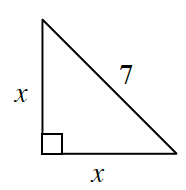### Home > INT3 > Chapter 7 > Lesson 7.2.5 > Problem7-108

7-108.

Examine the triangle below. Solve for $x$ twice, using two different methods. Clearly show your work for each method.This triangle is a $45^\circ-45^\circ-90^\circ$ triangle.

The Pythagorean Theorem can be used to solve for $x$.

$x ≈ 4.9$ units